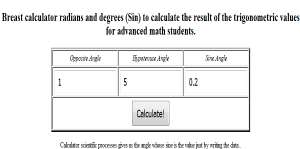﻿ Calculator for trigonometric sine Select Language

# Calculator for trigonometric functions sine Select language

Geometry calculator to calculate the sine free online web application cosines for mathematics

Select the language:

• English
• Español
• Free and without registration.Allows complex calculations using various trigonometric functions sine, cosine, for a variety of angles in degrees.

Calculator to perform more complex math operations, as well as sine, cosine, arc sine.br>

Home marzo de 2023 Terminos y Condiciones de uso  Cookies - Contacto